## Example Questions

← Previous 1

### Example Question #1 : How To Find The Midpoint Of A Line Segment

A line segment has endpoints (0,4) and (5,6). What are the coordinates of the midpoint?

(3,9)

(2.5,-5)

(0,6)

(0,4)

(2.5,5)

(2.5,5)

Explanation:

A line segment has endpoints (0,4) and (5,6). To find the midpoint, use the midpoint formula:

X: (x1+x2)/2 = (0+5)/2 = 2.5

Y: (y1+y2)/2 = (4+6)/2 = 5

The coordinates of the midpoint are (2.5,5).

### Example Question #1 : How To Find The Midpoint Of A Line Segment

Find the midpoint between (-3,7) and (5,-9)

(-1,-1)

(4,-1)

(1,-1)

(4,-8)

(1,-8)

(1,-1)

Explanation:

You can find the midpoint of each coordinate by averaging them.  In other words, add the two x coordinates together and divide by 2 and add the two y coordinates together and divide by 2.

x-midpoint = (-3 + 5)/2 = 2/2 = 1

y-midpoint = (7 + -9)/2 = -2/2 = -1

(1,-1)

### Example Question #1 : How To Find The Midpoint Of A Line Segment

Find the coordinates for the midpoint of the line segment that spans from (1, 1) to (11, 11).

(5, 5)

(6, 5)

(6, 6)

(7, 7)

(5, 6)

(6, 6)

Explanation:

The correct answer is (6, 6). The midpoint formula is ((x+ x2)/2),((y+ y2)/2) So 1 + 11 = 12, and 12/2 = 6 for both the x and y coordinates.

### Example Question #1 : How To Find The Midpoint Of A Line Segment

What is the midpoint between the points (–1, 2) and (3, –6)?

(1,2)

(–1,2)

(3,1)

(–2,–1)

(1,–2)

(1,–2)

Explanation:

midpoint = ((x1 + x2)/2, (y1 + y2)/2)

= ((–1 + 3)/2, (2 – 6)/2)

= (2/2, –4/2)

= (1,–2)

### Example Question #1 : Midpoint Formula

A line segment connects the points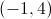and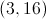. What is the midpoint of this segment?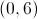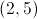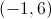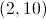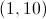Explanation:

To solve this problem you will need to use the midpoint formula: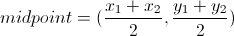Plug in the given values for the endpoints of the segment:and.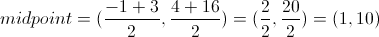### Example Question #6 : How To Find The Midpoint Of A Line Segment

What is the midpoint between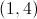and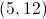?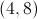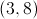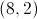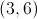Explanation:

The midpoint is the point halfway between the two endpoints, so sum up the coordinates and divide by 2: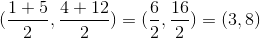### Example Question #7 : How To Find The Midpoint Of A Line Segment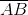has endpoints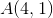and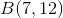What is the midpoint of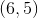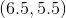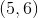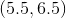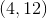Explanation:

The midpoint is simply the point halfway between the x-coordinates and halfway between the y-coordinates:

Sum the x-coordinates and divide by 2: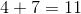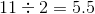Sum the y-coordinates and divide by 2: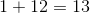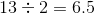Therefore the midpoint is (5.5, 6.5).

### Example Question #1 : How To Find The Midpoint Of A Line Segment

What is the mid point of a linear line segment that spans from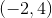to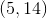?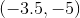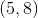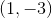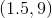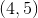Explanation:

To find the midpoint of a line segment, use this formula: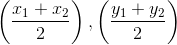Therefore, the midpoint of the given line segment is: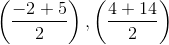### Example Question #9 : How To Find The Midpoint Of A Line Segment

Find the midpoint of the line segment with endpoints (1,3) and (5,7).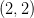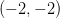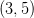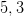Explanation:

To solve, simply use the midpoint formula as outlined below.

Given,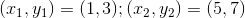Thus,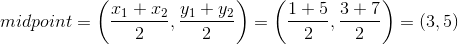### Example Question #10 : How To Find The Midpoint Of A Line Segment

Find the midpoint of a line segment with end points (1,3) and (11,3).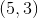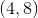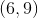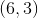Explanation:

To solve, simply realize you are on a horizantal line, so you just need to find the distance betweent he two x coordants and find half way between them. Thus,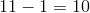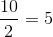Thus, the midpoint is at (1+5,3) which is (6,3).

← Previous 1

### All SAT Math Resources